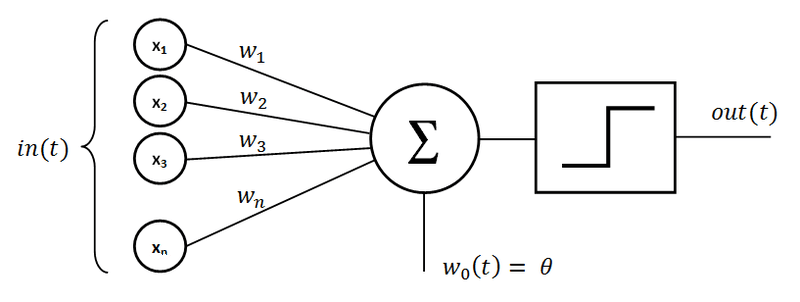Activation functions explained

I love this kind of featured images. It makes people think that you are so smart and that you’ve figured out life. They’re hilarious. It it makes the topic of the post seem like it’s really deep, pun intended.

If you’ve worked with neural networks even a little bit, you’ve probably come across the term activation function. You might be thinking, what is an activation function?

An activation function introduce non-linear properties to a neural network. Without activation functions, a neural network would be a linear polynomial. Machine learning does have models based on linear polynomials, linear regression models. Linear regression models can be used up to a point of complexity. As the data gets more and more complex and it  losses its linearity, linear regression models get useless. That’s where neural networks and activation functions come.The little square right before the output in the image above represents an activation function.

Most popular activation functons

At the time of writing this post, the most common activation functions are: sigmoid, Tanh and ReLu.

SigmoidSigmoid activation function is represented with an s-shaped curve. It normalizes a value between 0 and 1. Its mathematical representation is:.

This activation function has quite a few setbacks. Some of those are:

• slow convergence,
• hard to optimize.

While sigmoid is very popular, it is generally not recommended to use it. There are a couple other activation functions which perform much better, and we are going to cover a couple of those.

Its gradient is.

Hyperbolic tangent function

Hyperbolic tangent function, or Tanh for short, is an activation function which plots a value in the range between – 1 and 1.

Its mathematical representation is.Hyperbolic tangent function makes optimization much easier, but, like the sigmoid function, it suffers from the vanishing gradient problem.

Its derivative is.

Rectified Linear units function

Rectified Linear units function, or ReLu for short, is a very simple, but very efficient activation function.

It simply.

Simple as that. And because of its simplicity, it extremely computationally efficient. It needs to be used only with a hidden layer, and we use a softmax function for classification to compute the possibility.

Although, ReLu isn’t without problems ether. Sometimes, a node can “die” and become useless. If you encounter this kind of a problem, than consider using one of the following activation functions:

• Leaky ReLu – f(x) = {0.01x for x < 0;   x for x >= 0}
• Randomized leaky ReLu – f(a, x) = {axfor x < 0;   x for x >= 0}